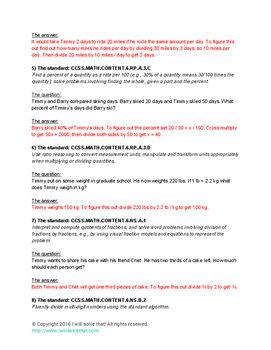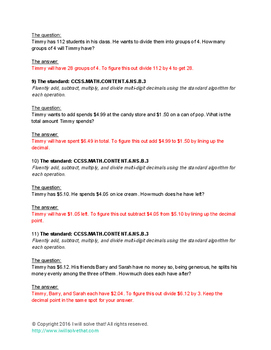# Math: Middle School "Bell Ringers"Grade Levels
6th - 8th
Subjects
Standards
Resource Type
Formats Included
• PDF
• EBooks
• Compatible withActivities
Pages
60 pages
Compatible with Easel Activities
This resource is compatible with Easel by TpT, a suite of digital tools you can use to make any lesson interactive and device-ready. Customize this activity and assign it to students, all from Easel. Easel is free to use! Learn more.

### Description

This eBook, "The Big Book of Timmy - Middle School Math," contains 122 bell ringers with answers and detailed explanations. Explore the adventures of Timmy as he tackles the Common Core math standards of 6th through 8th grade as he travels from the beaches of Cape Cod to the ski slopes of Vail, Colorado. Includes at least one question for each math standard of grades 6-8. This eBook provides problems that are perfect for warm up "bell ringers" for your students. This eBook is a 60 page PDF document.
Total Pages
60 pages
Answer Key
Included
Teaching Duration
N/A
Report this Resource to TpT
Reported resources will be reviewed by our team. Report this resource to let us know if this resource violates TpT’s content guidelines.

### Standards

to see state-specific standards (only available in the US).
Describe qualitatively the functional relationship between two quantities by analyzing a graph (e.g., where the function is increasing or decreasing, linear or nonlinear). Sketch a graph that exhibits the qualitative features of a function that has been described verbally.
Construct a function to model a linear relationship between two quantities. Determine the rate of change and initial value of the function from a description of a relationship or from two (𝘹, 𝘺) values, including reading these from a table or from a graph. Interpret the rate of change and initial value of a linear function in terms of the situation it models, and in terms of its graph or a table of values.
Interpret the equation 𝘺 = 𝘮𝘹 + 𝘣 as defining a linear function, whose graph is a straight line; give examples of functions that are not linear. For example, the function 𝘈 = 𝑠² giving the area of a square as a function of its side length is not linear because its graph contains the points (1,1), (2,4) and (3,9), which are not on a straight line.
Compare properties of two functions each represented in a different way (algebraically, graphically, numerically in tables, or by verbal descriptions). For example, given a linear function represented by a table of values and a linear function represented by an algebraic expression, determine which function has the greater rate of change.
Understand that a function is a rule that assigns to each input exactly one output. The graph of a function is the set of ordered pairs consisting of an input and the corresponding output.

### Questions & Answers

Teachers Pay Teachers is an online marketplace where teachers buy and sell original educational materials.

More About Us